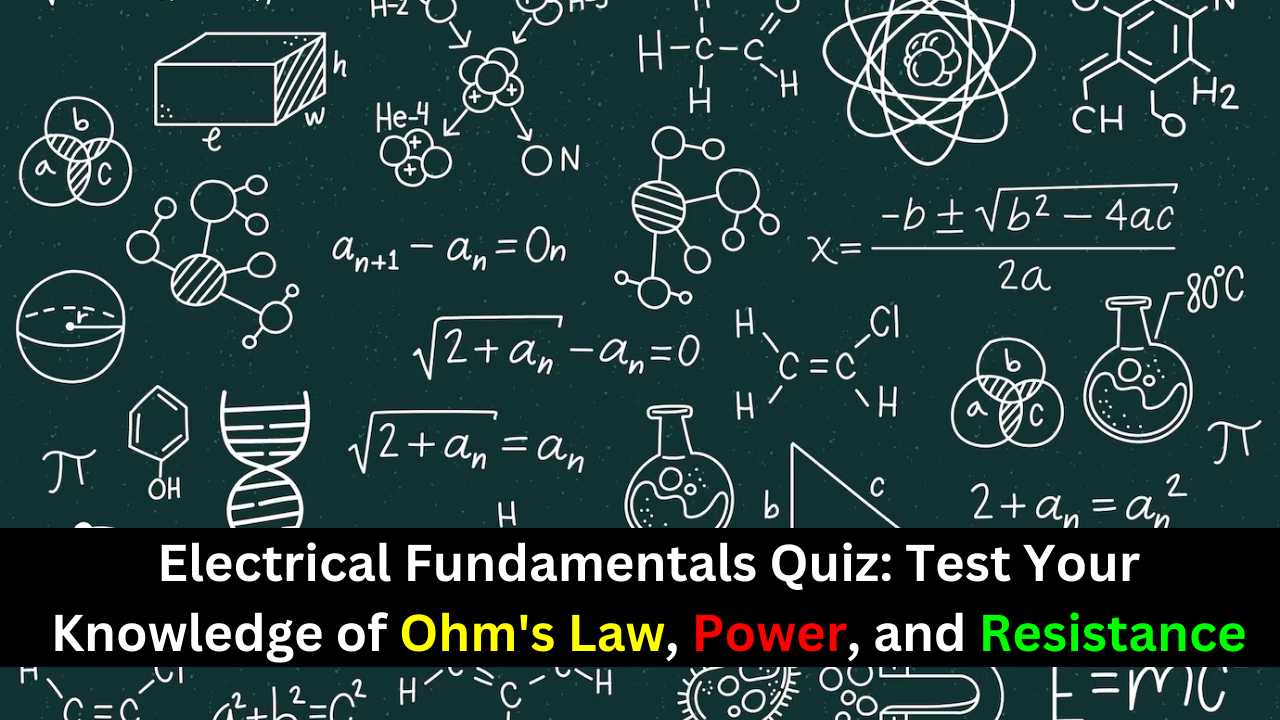## Electrical Fundamentals Quiz: Test Your Knowledge of Ohm’s Law, Power, and Resistance## Ohm’s law states that

(a) current equals voltage times resistance
(b) voltage equals current times resistance
(c) resistance equals current divided by voltage
(d) voltage equals current squared times resistance
Answer: (b) voltage equals current times resistance

(a) triple
(b) halve
(c) double
(d) not change

(a) 10 A
(b) 0.5 A
(c) 200 A
(d) 2 A

(a) 100 V
(b) 0.1 V
(c) 10 kV
(d) 10 V

(a) 53.2 V
(b) 1.175 mV
(c) 18.8 V
(d) 1.175 V

### Power can be defined as

(a) energy
(b) heat
(c) the rate at which energy is used
(d) the time required to use energy
Answer: (c) the rate at which energy is used

### For 10 V and 50 mA, the power is

(a) 500 mW
(b) 0.5 W
(c) (d) answers (a), (b), and (c)

(a) 1 W
(b) 10 W
(c) 100 mW
(d) 1 mW

(a) 0.25 W
(b) 1 W
(c) 2 W
(d) 5 W

(a) overheated
(b) shorted
(c) open
(d) reversed

(a) 2 mA
(b) 1 mA
(c) 4 mA
(d) 0.4 mA

### To measure the current out of the third resistor in a circuit consisting of four series resistors, an ammeter can be placed

(a) between the third and fourth resistors
(b) between the second and third resistors
(c) at the positive terminal of the source
(d) at any point in the circuit
Answer: (a) between the third and fourth resistors

### When a third resistor is connected in series with two series resistors, the total resistance

(a) remains the same
(b) increases
(c) decreases
(d) increases by one-third

### When one of four series resistors is removed from a circuit and the circuit reconnected, the current

(a) decreases by the amount of current through the removed resistor
(b) decreases by one-fourth
(d) increases
Answer: (a) decreases by the amount of current through the removed resistor

### While putting four 1.5 V batteries in a flashlight, you accidentally put one of them in backward. The light will be

(a) brighter than normal
(b) dimmer than normal
(c) off
(d) the same

### If you measure all the voltage drops and the source voltage in a series circuit and add them together, taking into consideration the polarities, you will get a result equal to

(a) the source voltage
(b) the total of the voltage drops
(c) zero
(d) the total of the source voltage and the voltage drops

### There are six resistors in a given series circuit, and each resistor has 5 V dropped across it. The source voltage is

(a) 5 V
(b) 30 V
(c) dependent on the resistor values
(d) dependent on the current

### Which of the following series combinations dissipates the most power when connected across a 100 V source?

(a) one resistor
(b) two resistors
(c) three resistors
(d) four resistors

(a) 1 W
(b) 5 W
(c) 0.5 W
(d) 0.2 W

### When you connect an ammeter in a series resistive circuit and turn on the source voltage, the meter reads zero. You should check for

(a) a broken wire
(b) a shorted resistor
(c) an open resistor
(d) both (a) and (c)
Answer: (d) both (a) and (c)

### While checking out a series resistive circuit, you find that the current is higher than it should be. You should look for

(a) an open circuit
(b) a short
(c) a low resistor value
(d) both (b) and (c)
Answer: (d) both (b) and (c)

### The output of a certain voltage divider is 9 V with no load. When a load is connected, the output voltage

(a) increases
(b) decreases
(c) remains the same
(d) becomes zero

### When a load resistance is connected to the output of a voltage-divider circuit, the current drawn from the source

(a) decreases
(b) increases
(c) remains the same
(d) is cut off

### The output voltage of a balanced Wheatstone bridge is

(a) equal to the source voltage
(b) equal to zero
(c) dependent on all of the resistor values in the bridge
(d) dependent on the value of the unknown resistor

### The primary method of analyzing a circuit with two or more voltage sources is usually

(a) Thevenin’s theorem
(b) Ohm’s law
(c) superposition
(d) Kirchhoff’s law

### A Thevenin equivalent circuit consists of

(a) a voltage source in series with a resistance
(b) a voltage source in parallel with a resistance
(c) a current source in parallel with a resistance
(d) two voltage sources and a resistance
Answer: (a) a voltage source in series with a resistance

### You are measuring the voltage at a given point in a circuit that has very high resistance values and the measured voltage is a little lower than it should be. This is possible because of

(a) one or more of the resistance values being off